Example Question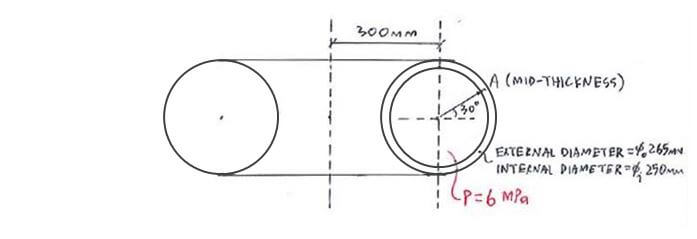Click below to show answer. X

Step 1: Get values for ro, rΦ, rθ, Φ, p, t Step 1: Get values for ro, rΦ, rθ, Φ, p, t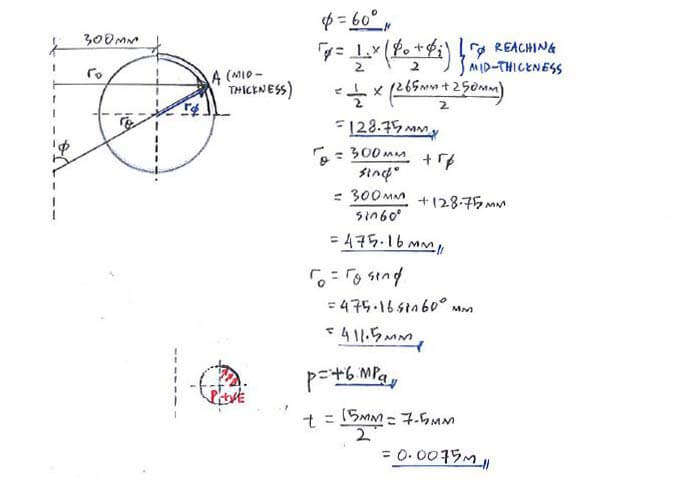Step 2: [+↑ΣFy = 0], find σΦ Step 2: [+↑ΣFy = 0], find σΦ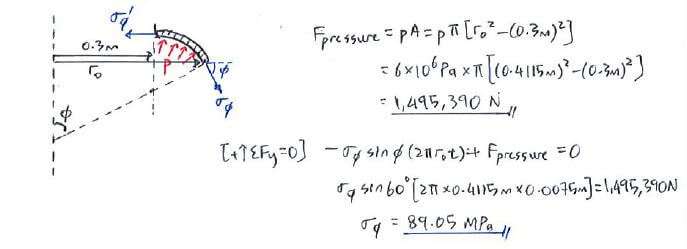Step 3: Membrane stress equation, find σθ Step 3: Membrane stress equation, find σθ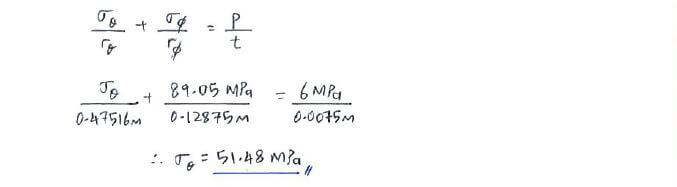Step 4: Final answer for σθ, σΦ, σr Step 4: Final answer for σθ, σΦ, σr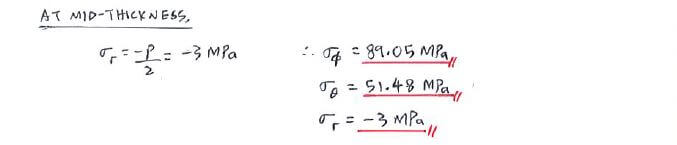Useful formula: Useful formula:Now that you've mastered this question, you can try Question 1.